Updating search results...

# 59 Results

View
Selected filters:
• cl5lesson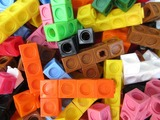Conditional Remix & Share Permitted
CC BY-NC-SA
Rating
0.0 stars

In this lesson students evaluate expressions and consider how the Order of Operations changes the value of the expressions.&nbsp;

Subject:
Mathematics
Material Type:
Activity/Lab
Lesson Plan
Author:
Drew Polly
07/27/2020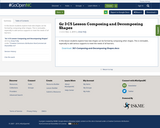Conditional Remix & Share Permitted
CC BY-NC-SA
Rating
0.0 stars

In this lesson students explore how new shapes can be formed by composing other shapes. This is remixable , especially to add various supports to meet the needs of all learners.

Subject:
Mathematics
Material Type:
Activity/Lab
Lesson Plan
11/06/2019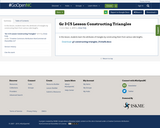Conditional Remix & Share Permitted
CC BY-NC-SA
Rating
0.0 stars

In this lesson, students learn the attributes of triangles by constructing them from various side lengths.

Subject:
Mathematics
Material Type:
Activity/Lab
Lesson Plan
11/18/2019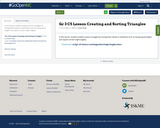Conditional Remix & Share Permitted
CC BY-NC-SA
Rating
0.0 stars

In this lesson, students explore various triangles by sorting them based on attributes such as having equal lengths and square corners (right angles).

Subject:
Mathematics
Material Type:
Activity/Lab
Lesson Plan
11/18/2019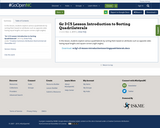Conditional Remix & Share Permitted
CC BY-NC-SA
Rating
0.0 stars

In this lesson, students explore various quadrilaterals by sorting them based on attributes such as opposite sides having equal lengths and square corners (right angles).

Subject:
Mathematics
Material Type:
Activity/Lab
Lesson Plan
11/18/2019Conditional Remix & Share Permitted
CC BY-NC-SA
Rating
0.0 stars

In this lesson, students identify and explain the attributes of a quadrilateral and use defining attributes (number of sides, angles) to name quadrilaterals.

Subject:
Mathematics
Material Type:
Activity/Lab
Lesson Plan
11/18/2019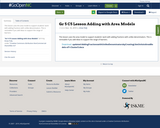Conditional Remix & Share Permitted
CC BY-NC-SA
Rating
0.0 stars

This lesson uses the area model to support students' work with adding fractions with unlike denominators. This is remixable if you add ideas to support the range of learners.

Subject:
Mathematics
Material Type:
Activity/Lab
Lesson Plan
11/16/2019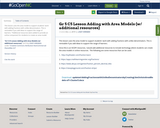Conditional Remix & Share Permitted
CC BY-NC-SA
Rating
0.0 stars

This lesson uses the area model to support students' work with adding fractions with unlike denominators. This is remixable if you add ideas to support the range of learners.

*Additional resources were added to provide an online component for students to create an area model.

Subject:
Mathematics
Material Type:
Activity/Lab
Lesson Plan
12/02/2019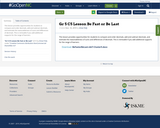Conditional Remix & Share Permitted
CC BY-NC-SA
Rating
0.0 stars

This lesson provides opportunities for students to compare and order decimals, add and subtract decimals, and estimate the reasonableness of sums and differences of decimals. This is remixable if you add additional supports for the range of learners.

Subject:
Mathematics
Material Type:
Activity/Lab
Lesson Plan
11/16/2019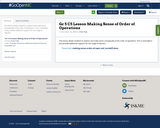Conditional Remix & Share Permitted
CC BY-NC-SA
Rating
0.0 stars

This lesson allows students to explore and make sense conceptually of the order of operations. This is remixable if you provide additional supports for the range of learners.

Subject:
Mathematics
Material Type:
Activity/Lab
Lesson Plan
11/16/2019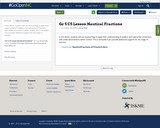Conditional Remix & Share Permitted
CC BY-NC-SA
Rating
0.0 stars

In this lesson, students will use nautical flags to apply their understanding of addition and subtraction of fractions with unlike denominators within context. This is remixable if you provide additional supports for the range of learners.

Subject:
Mathematics
Material Type:
Activity/Lab
Lesson Plan
11/16/2019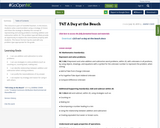Conditional Remix & Share Permitted
CC BY-NC-SA
Rating
0.0 stars

This resource is part of Tools4NCTeachers.

In this lesson, students solve a word problem using a strategy of choice and share the strategy to develop the concept of representing and solving problems involving addition and subtraction within 20. This problem type will likely provide an opportunity to explore the commutative property with students. This lesson format may be used with any problem type appropriate for the grade.

Subject:
Mathematics
Material Type:
Lesson
Lesson Plan
11/06/2019Unrestricted Use
CC BY
Rating
0.0 stars

This resource is from Tools4NCTeachers.&nbsp;In this lesson, students develop strategies for adding 3-digit numbers, with a focus on adding in chunks.&nbsp; Students build this strategy of adding in chunks by mentally adding 10 and 100 to numbers, and adding on the number line.&nbsp;Remix this lesson to include extension activities such as math stations.

Subject:
Mathematics
Material Type:
Activity/Lab
Lesson
Lesson Plan
Author:
DAWNE COKER
06/23/2020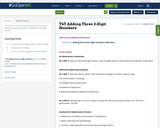Conditional Remix & Share Permitted
CC BY-NC-SA
Rating
0.0 stars

This resource is part of Tools4NCTeachers.

In this lesson, students explore approaches for adding three two-digit numbers.

Subject:
Mathematics
Material Type:
Lesson
Lesson Plan
11/06/2019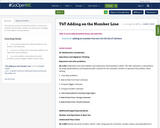Conditional Remix & Share Permitted
CC BY-NC-SA
Rating
0.0 stars

This resource is part of Tools4NCTeachers.

In this lesson students use the open number line and various strategies to solve addition story problems.

Subject:
Mathematics
Material Type:
Lesson
Lesson Plan
11/06/2019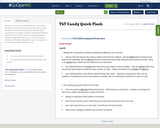Conditional Remix & Share Permitted
CC BY-NC-SA
Rating
0.0 stars

This resource is part of Tools4NCTeachers.

This lesson should occur after the Partner Quick Flash lesson, which introduces conceptual subitizing. Candy Quick Flash continues to encourage conceptual subitizing an efficient way to find "how many".

Notes:
Perceptual subitizing: instantly recognizing "how many" in a set
Conceptual subitizing: efficiently finding "how many" by recognizing and combining subgroups within a set

Subject:
Mathematics
Material Type:
Lesson
Lesson Plan
11/06/2019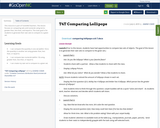Conditional Remix & Share Permitted
CC BY-NC-SA
Rating
0.0 stars

This resource is part of Tools4NCTeachers.

This lesson focuses on comparing sets and numerals using terms greater than, less than, and equal to. The main goal is for students to generate their own sets to compare to a given set.

Subject:
Mathematics
Material Type:
Lesson
Lesson Plan
11/06/2019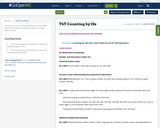Conditional Remix & Share Permitted
CC BY-NC-SA
Rating
0.0 stars

This resource is part of Tools4NCTeachers.

In this lesson students participate in activities focused on counting objects by tens.

Subject:
Mathematics
Material Type:
Lesson
Lesson Plan
11/06/2019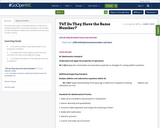Conditional Remix & Share Permitted
CC BY-NC-SA
Rating
0.0 stars

This resource is part of Tools4NCTeachers.

In this lesson, students explore the communitive property in the context of a word problem to determine if students have the same number of items

Subject:
Mathematics
Material Type:
Lesson
Lesson Plan
11/06/2019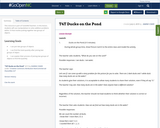Conditional Remix & Share Permitted
CC BY-NC-SA
Rating
0.0 stars

This resource is part of Tools4NCTeachers.

In this lesson, students will use manipulatives to solve addition problems within 10 that involve putting together two groups of objects.

Subject:
Mathematics
Material Type:
Lesson
Lesson Plan